# Degrees

Have you ever observed Carpenter's square?

This is how it looks!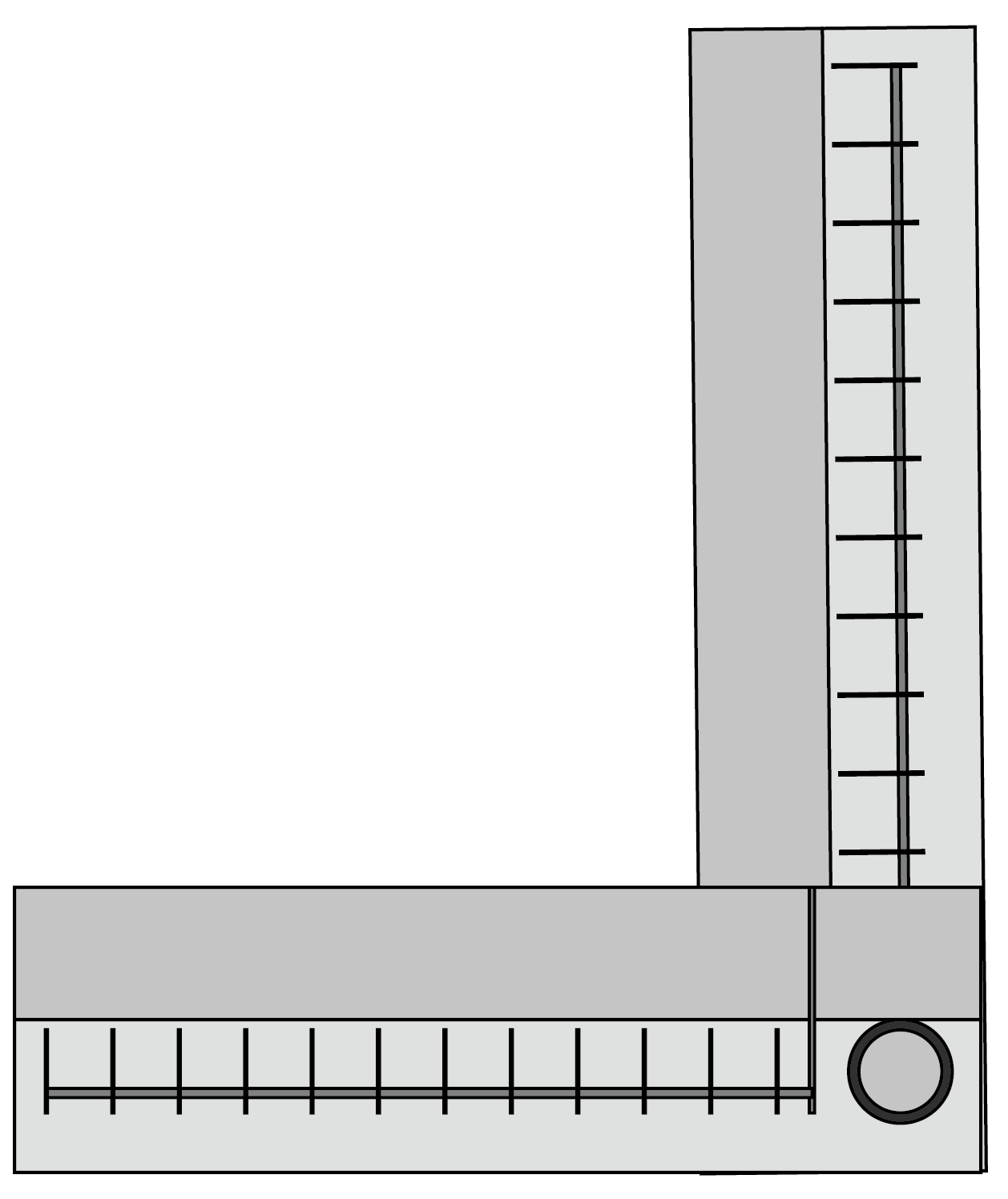The carpenter uses it to check right angles.

Mia also wants to make a similar angle tester.Look at the steps she followed.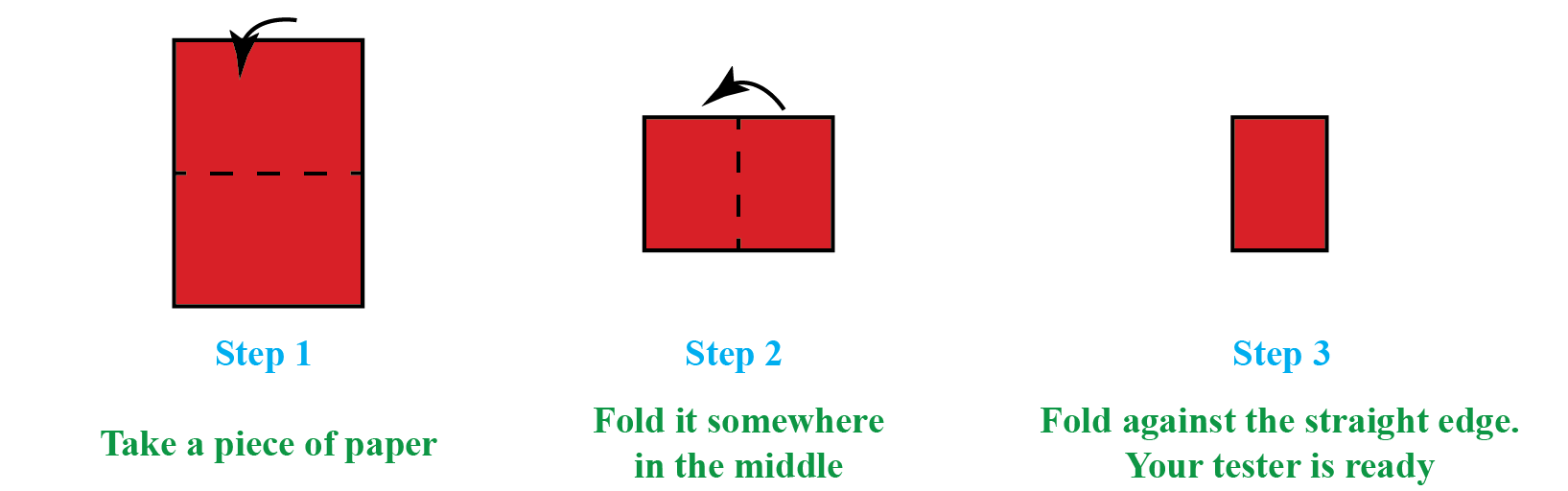She uses this right-angle tester to test right angles, acute angles, or obtuse angles.  But she wondered that this does not give her a precise comparison.She cannot find which one among the two obtuse angles is greater.

So, in order to get an accurate comparison, we measure the angles and angles are measured in degrees!

In this mini-lesson, we will learn about the definition of degree, the degree symbol, degree angle, degree to radians conversion, and also about protractor. Check-out the interactive simulations to know more about the lesson and try your hand at solving a few interesting practice questions at the end of the page.

## Lesson Plan

 1 Definition of Degree 2 Thinking Out of the Box! 3 Important Notes on Degrees 4 Solved Examples on Degrees 5 Interactive Questions on Degrees

## Definition of Degree

Angles are measured in degrees.

One revolution is divided into 360 equal parts and each part is called a degree.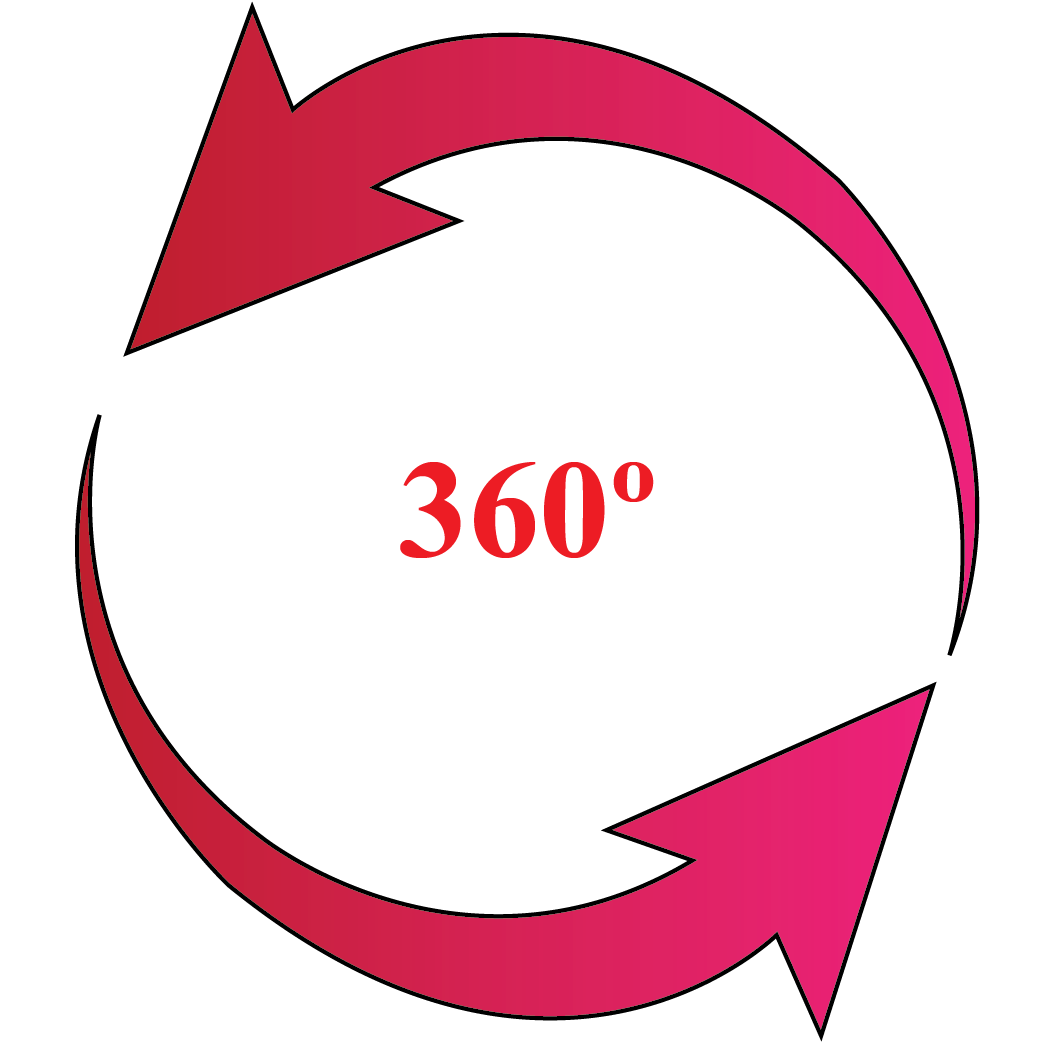### Degree Symbol

We usually denote a degree with a small circle $$^\circ$$.

For example, $$45^\circ$$ means 45 degrees.

So, now you know how to use the degree symbol.

### The Degree to Radians Conversion

Apart from degrees angles are also measured in radians.

The international bureau of weights and measures specify rad as a symbol of the radians.

You can convert the degree to radians by using formula:

 \begin{align}1\:\text{degree} = \frac{\pi}{180}\mathrm{\;rad}\end{align}Think Tank
1. How many degrees are there in half a revolution?
2. How many right angles make $$360^{\circ}$$?

## How to Measure Degrees?

### Process

Follow the following steps to measure the angle in degrees.

• Keep the protractor in such a way that its midpoint coincides with vertex $$B$$ of the angle.
• Adjust the proctor so that $$\overline{BC}$$ lies along the straight-edge of the protractor.
• Read the scale which has the $$0^{\circ}$$ mark coinciding with the straight-edge.
• The mark $$\overline{BC}$$ on the curved edge gives the degree measure.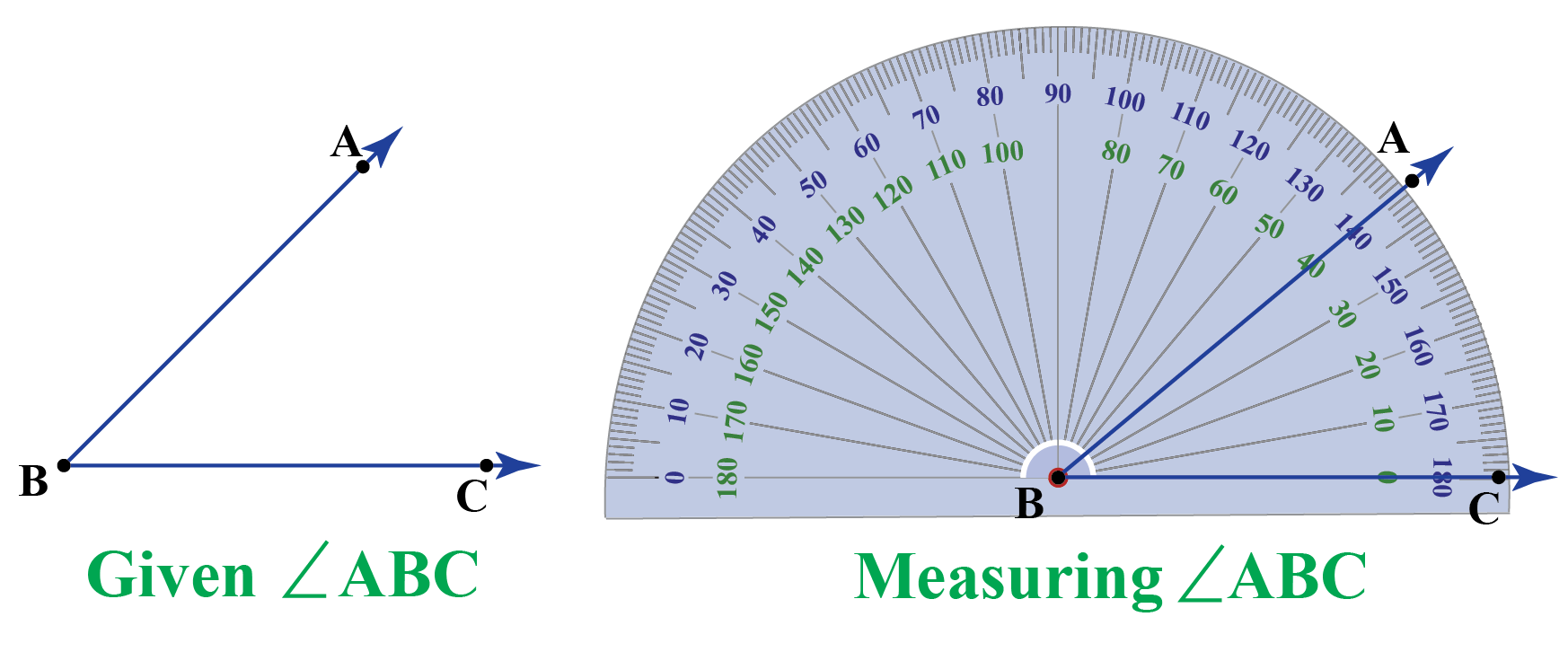## Measuring Angles in Degrees

Using a protractor is the best way to measure angles.

The curved edge of the protractor is divided into 180 equal parts.

There are two sets of numbers on a protractor:

• One in a clockwise direction
• Another in a counter-clockwise direction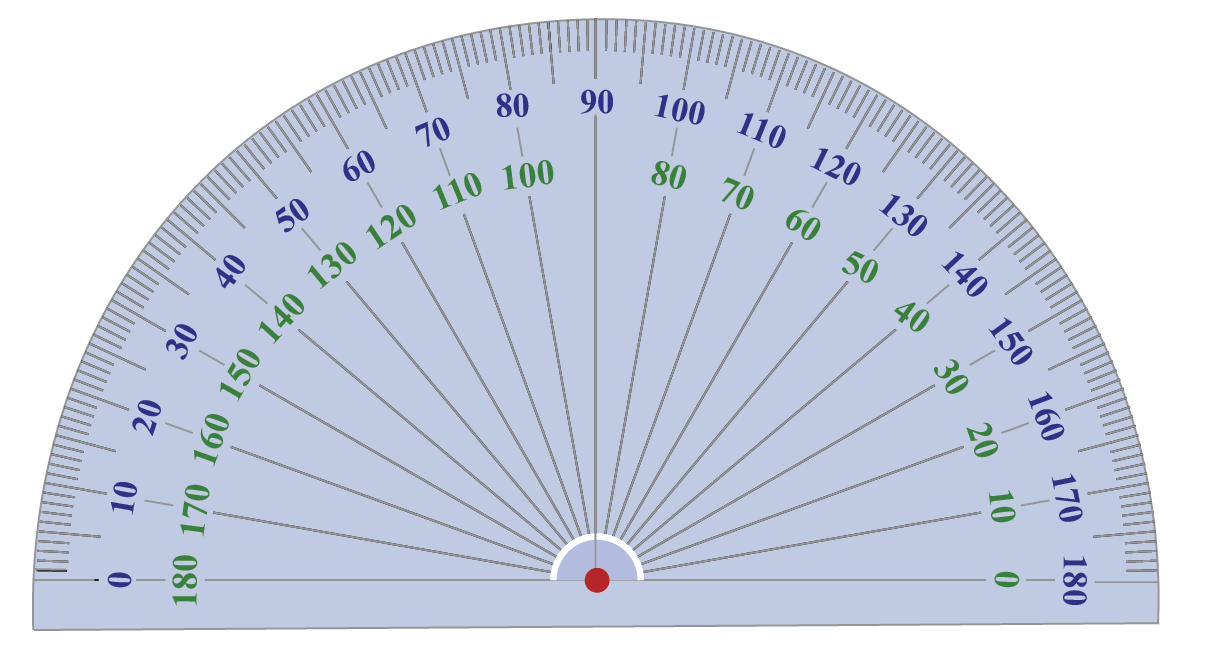If you observe closely, a protractor tool has degrees marked from $$0^{\circ}$$ to $$180^{\circ}$$ from left to right in the outer edge and from $$180^{\circ}$$ to $$0^{\circ}$$ in the inner part.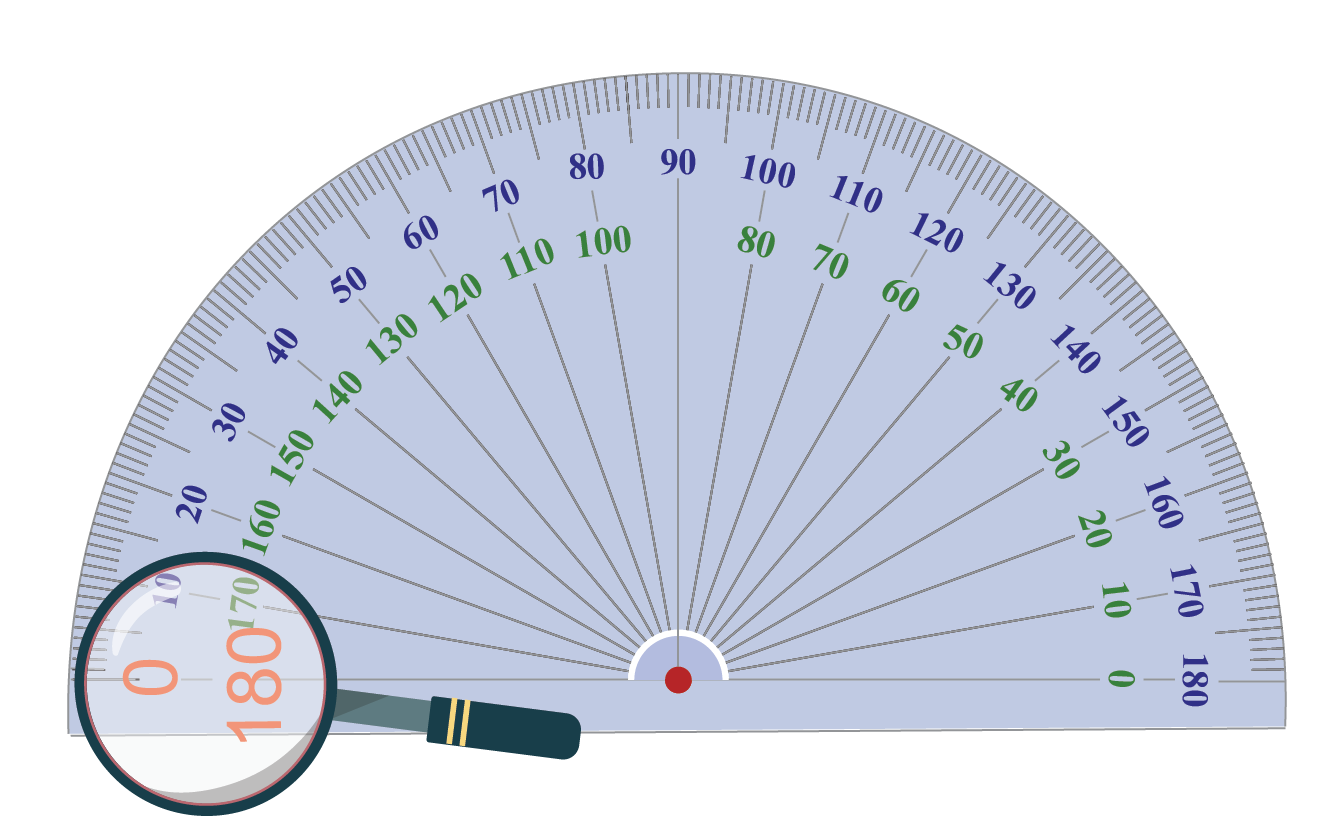The inner readings and outer reading supplements each other. i.e., they add up to form a complete $$180^ \circ$$ degree angle.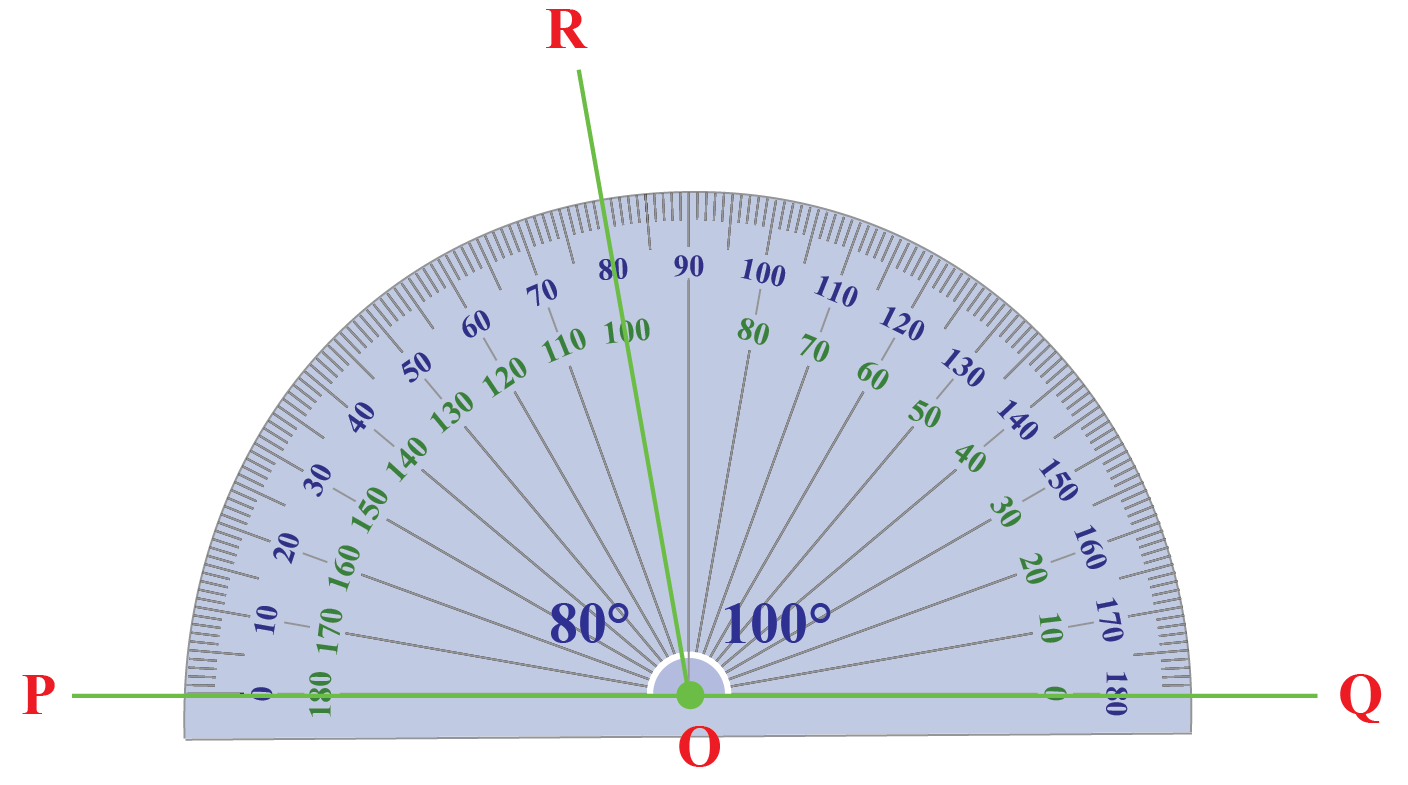If the angle to be measured is at the left part of the protractor, we need to use the outer reading of the protractor.

$\angle\text{POR}=80^{\circ}$

If the angle to be measured is at the right part of the protractor, we need to use the inner readings of the protractor.

$\angle\text{QOR}=100^{\circ}$

Let’s try and see if we can find out what type of angle is $$\angle{AOB}$$ given below.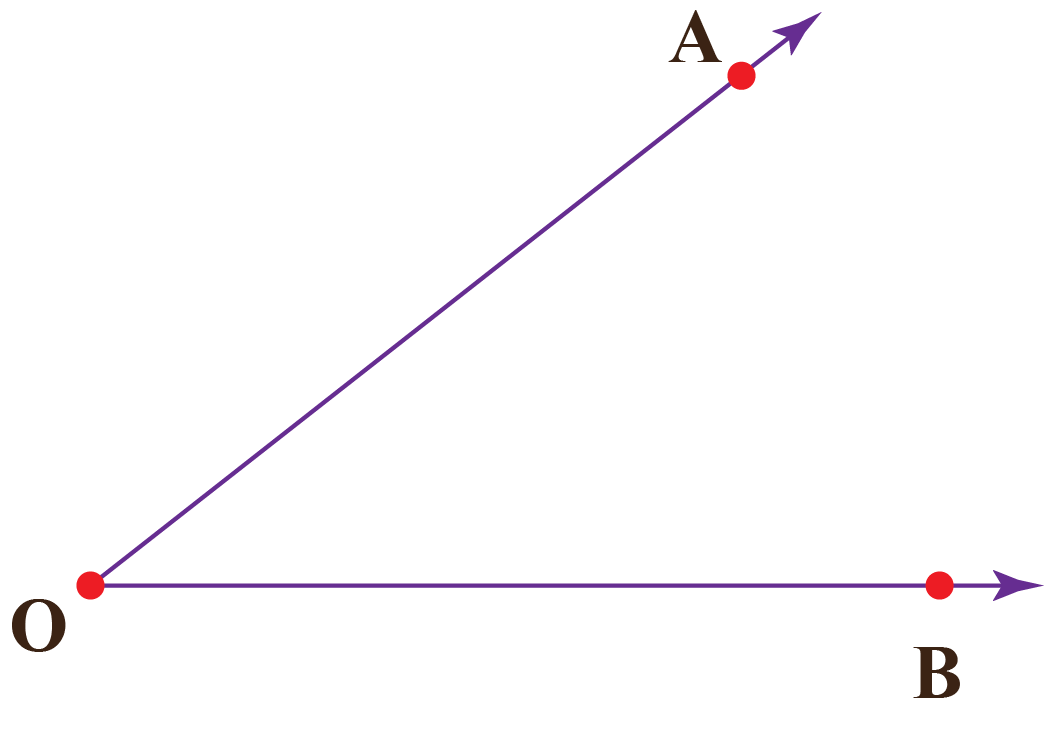Doesn't it look like an acute angle?

This means that its measure is greater than $${0^\circ}$$ and less than $${90^\circ}$$ degree angle.

Now let’s try to find its exact measure with the help of a protractor.

Step 1

Align the protractor with the ray OB as shown below.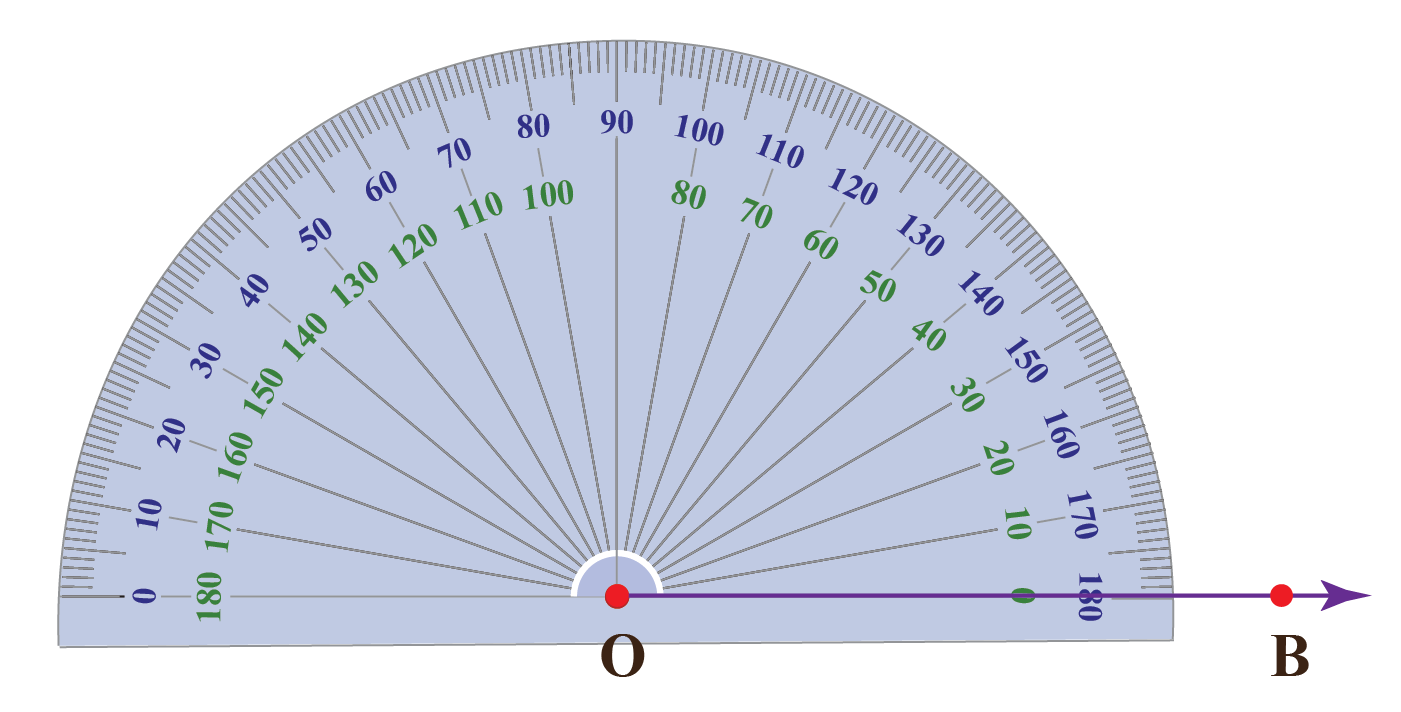Start reading from the $${0^\circ}$$ mark on the bottom-right of the protractor.

Step 2

Measure the angle using the inner readings on the lower arc (inner readings)  of the protractor.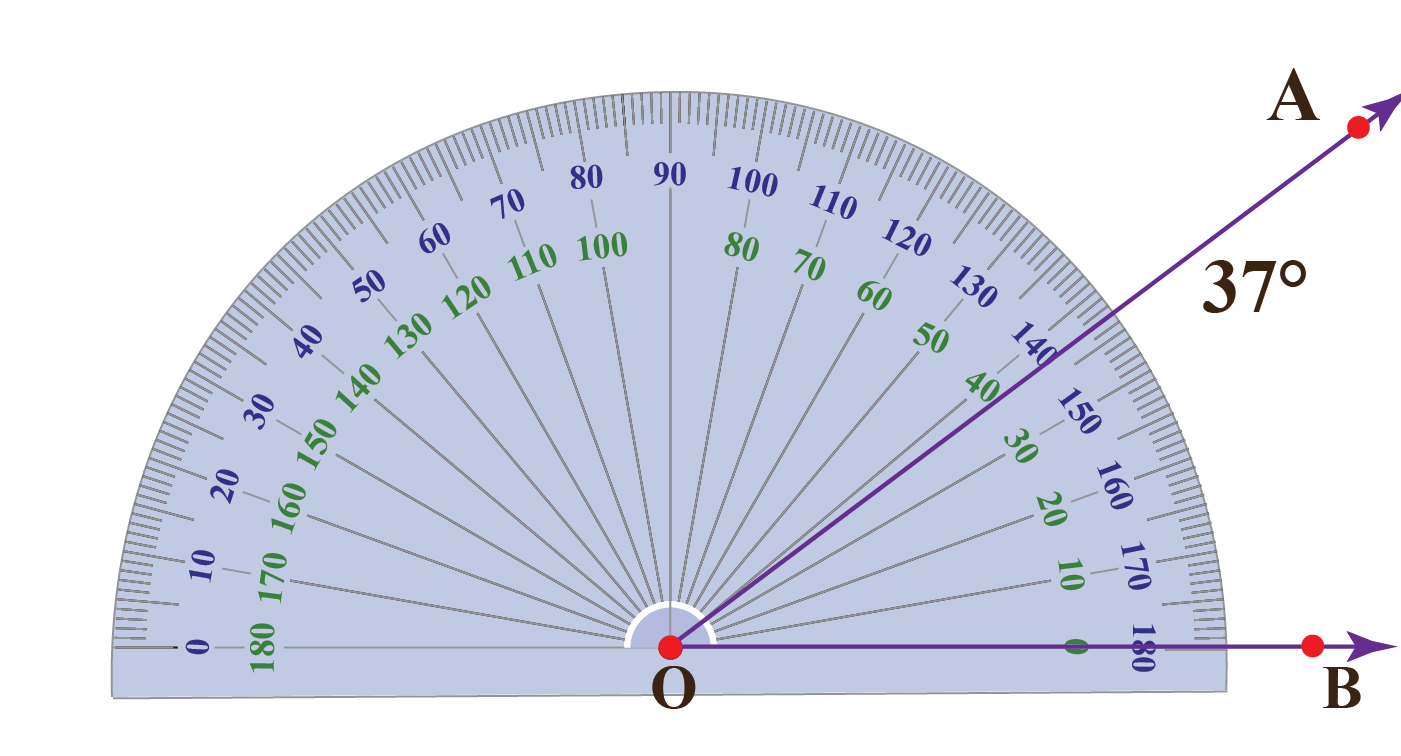$$\angle{AOB}=37^\circ$$

Click on the new angle button to generate new angles and check your answer.You can try measuring various angles in this simulation by entering the degree value and check if it is correct.

## Solved Examples on Degrees

 Example 1

Use a protractor and verify if the angle beneath the staircase is $$140^\circ$$.Solution

We can use a protractor and measure the given angle.

Place the protractor such that $$M$$ coincides with the midpoint of the protractor.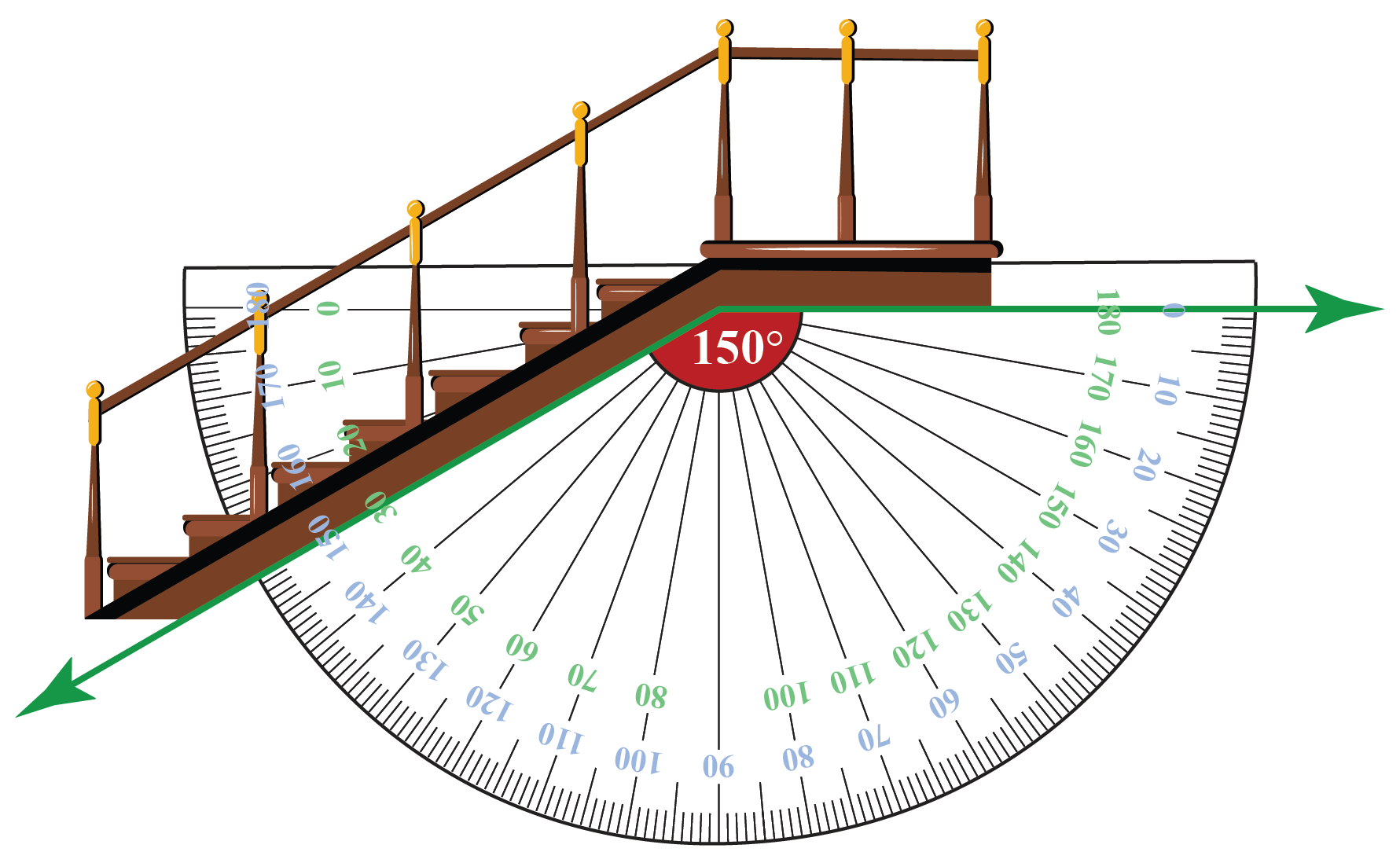If you carefully observe in the figure, a 150-degree angle appears on the lower (outer) arc of the protractor.

 $$\therefore$$ The angle formed is $$150^\circ$$ and not $$140^\circ$$.
 Example 2

In a triangle $$\Delta ABC$$, use a protractor and measure the degree angle $$\angle{CAB}$$.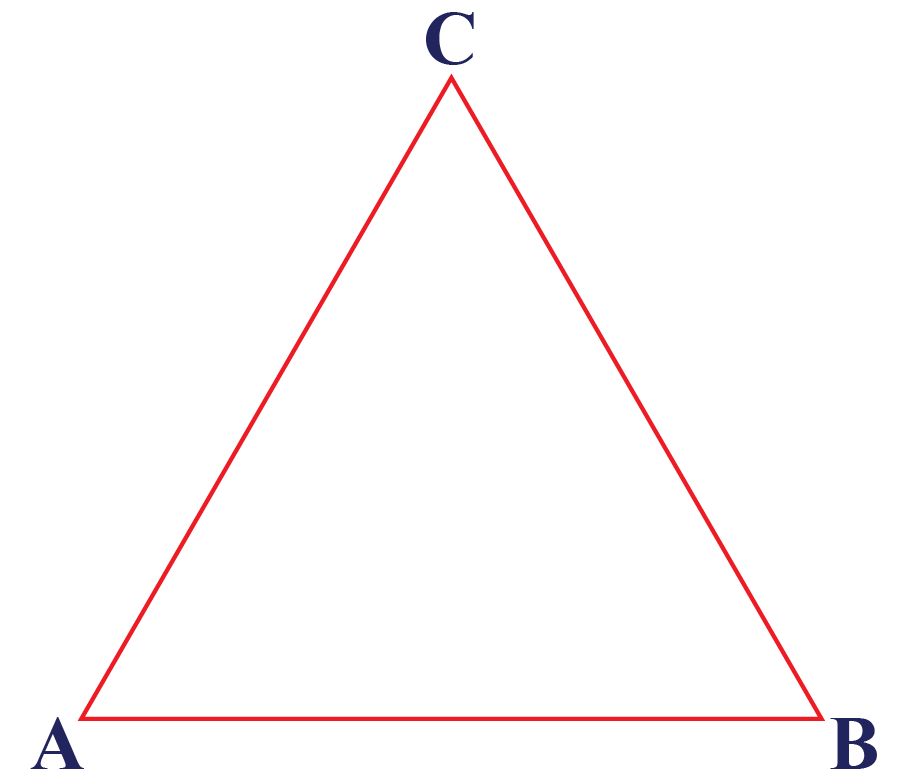Solution

Place the protractor baseline along the line AB and the center of the protractor at the vertex A.

Observe the reading in the protractor which overlaps with line AC.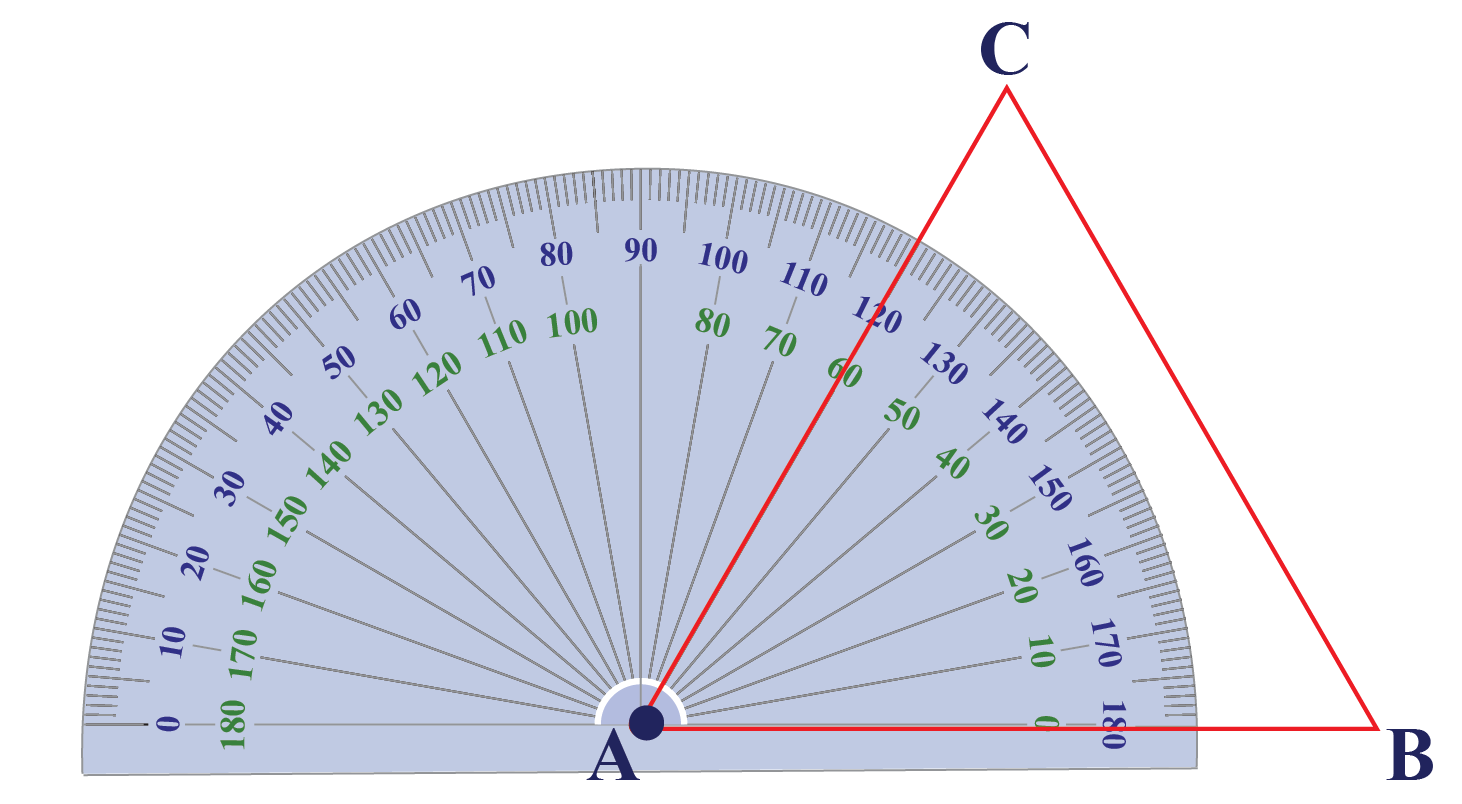The angle which is to be measured is at the right part of the protractor.

Therefore, use the inner readings of the protractor.

 $$\therefore$$ $$\angle{CAB}=60^{\circ}$$
 Example 3

Emma and James are cutting papers with the help of scissors to build some designs.

James observes that during the cutting process scissors make an angle.He asked Emma what is the angle in degree formed between the two arms of the given pair of scissors?

Can you help her?

Solution

Place the protractor along one arm and measure the angle.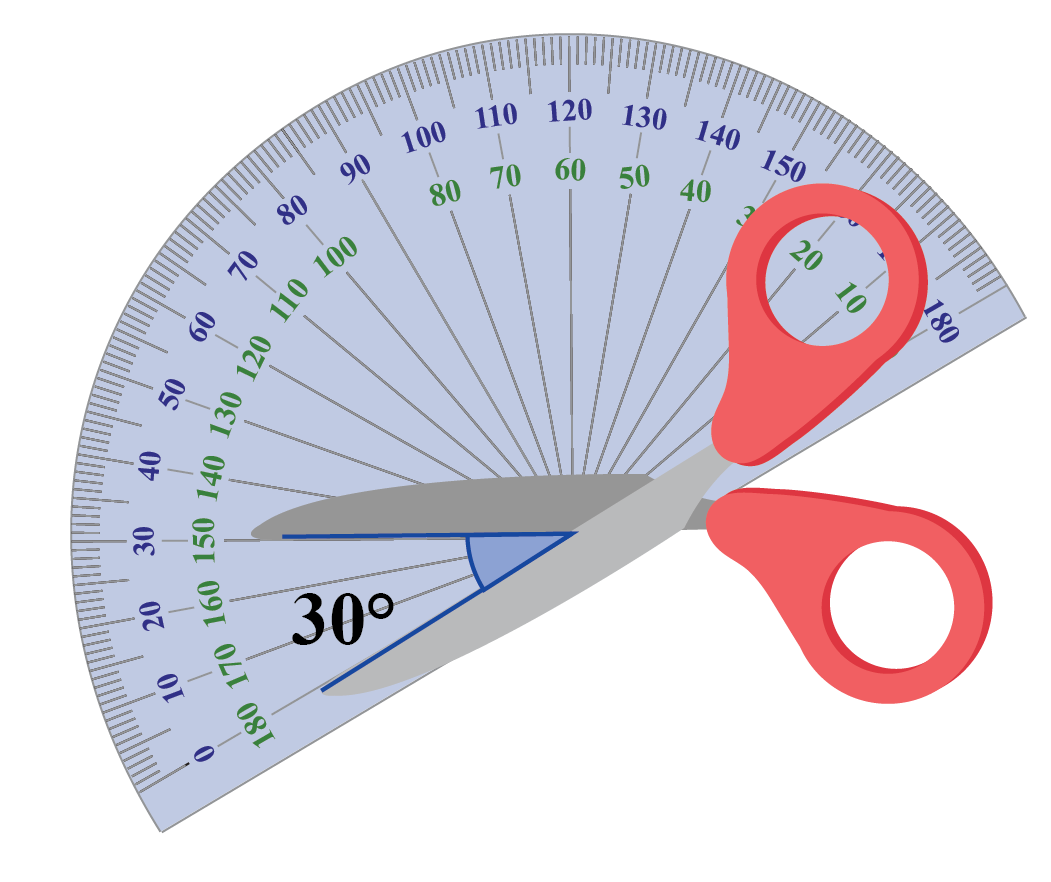The angle which is to be measured is at the left part of the protractor.

Therefore, use the outer readings of the protractor.

 $$\therefore$$ $$\text{Angle formed} = 30^{\circ}$$
 Example 4

Eight slices are cut from a circular pizza.

Determine the angle formed by a single slice in degrees.Solution

Place the protractor along one edge of the pizza slice and measure the angle.The angle which is to be measured is at the right part of the protractor.

Therefore, use the inner readings of the protractor.

 $$\therefore$$ $$\text{Angle formed} = 45^{\circ}$$
 Example 5

Help Jack to convert the 60-degree angle into radians.

Solution

The formula for the conversion of degree into radians is, $$1\;\text{degree}= \frac{\pi}{180}\;\mathrm{rad}$$.

Now multiply 60 on both the sides,

\begin{aligned}1\;\text{degree}&=\frac{\pi}{180}\;\mathrm{rad}\\60\;\text{degree}&=\frac{\pi}{180}\times60^{\circ}\;\mathrm{rad}\\&=\frac{\pi}{3}\;\mathrm{rad}\\&=1.047\;\mathrm{rad}\end{aligned}

 $$\therefore$$ $$60\;\text{degrees}=1.047\;\mathrm{rad}$$

## Interactive Questions on Degrees

Here are a few activities for you to practice. Select/Type your answer and click the "Check Answer" button to see the result.

## Let's Summarize

The mini-lesson targeted the fascinating concept of degrees and measuring angles. The math journey around degrees starts with what a student already knows, and goes on to creatively crafting a fresh concept in the young minds. Done in a way that not only it is relatable and easy to grasp, but also will stay with them forever. Here lies the magic with Cuemath.

At Cuemath, our team of math experts is dedicated to making learning fun for our favorite readers, the students!

Through an interactive and engaging learning-teaching-learning approach, the teachers explore all angles of a topic.

Be it worksheets, online classes, doubt sessions, or any other form of relation, it’s the logical thinking and smart learning approach that we, at Cuemath, believe in.

## 1. What is 1 degree in radians?

\begin{aligned}1\;\text{degree}&=\frac{\pi}{180}\;\text{radians}\\&=0.0174533\;\text{radians}\end{aligned}

## 2. What is a protractor?

A protractor is a tool that helps us to measure angles in degrees.

More Important Topics
Numbers
Algebra
Geometry
Measurement
Money
Data
Trigonometry
Calculus